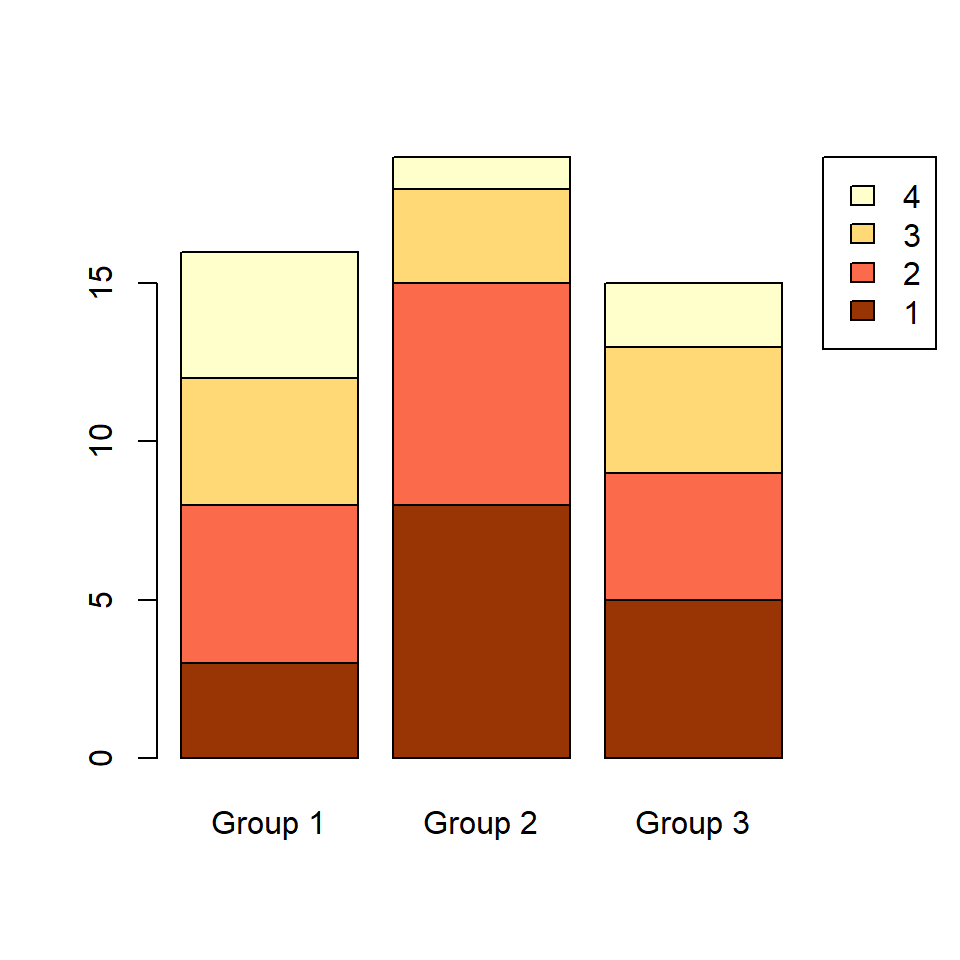# Stacked bar graph in R

## Data

Consider the data of the following table, which shows the count of the values of the variable `x` within three different groups.

``````# Data
set.seed(1)
x <- sample(1:4, size = 50, replace = TRUE)
g <- sample(c("Group 1", "Group 2", "Group 3"),
size = 50, replace = TRUE)

# Create a table from your data
tabl <- table(x, g)
tabl``````
x Group 1 Group 2 Group 3
1 3 8 5
2 5 7 4
3 4 3 4
4 4 1 2

## Stacked bar chart

In order to create a stacked bar chart, also known as stacked bar graph or stacked bar plot, you can use `barplot` from base R graphics. Note that you can add a title, a subtitle, the axes labels with the corresponding arguments or remove the axes setting `axes = FALSE`, among other customization arguments.

``````barplot(tabl,
main = "Stacked bar chart",
sub = "Subtitle",
xlab = "X-lab",
ylab = "Y-lab",
axes = TRUE)``````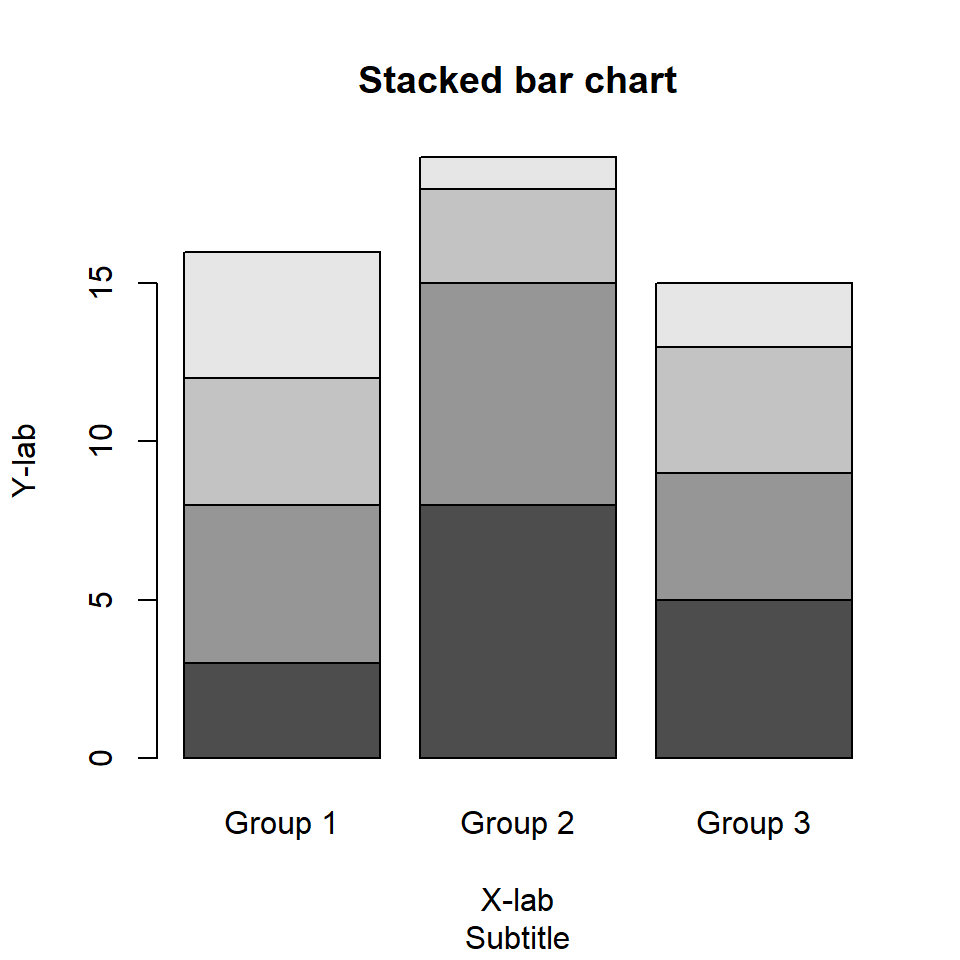Horizontal bar chart

If you prefer a horizontal stacked bar chart rather than vertical set `horiz = TRUE`.

``````barplot(tabl,
horiz = TRUE)``````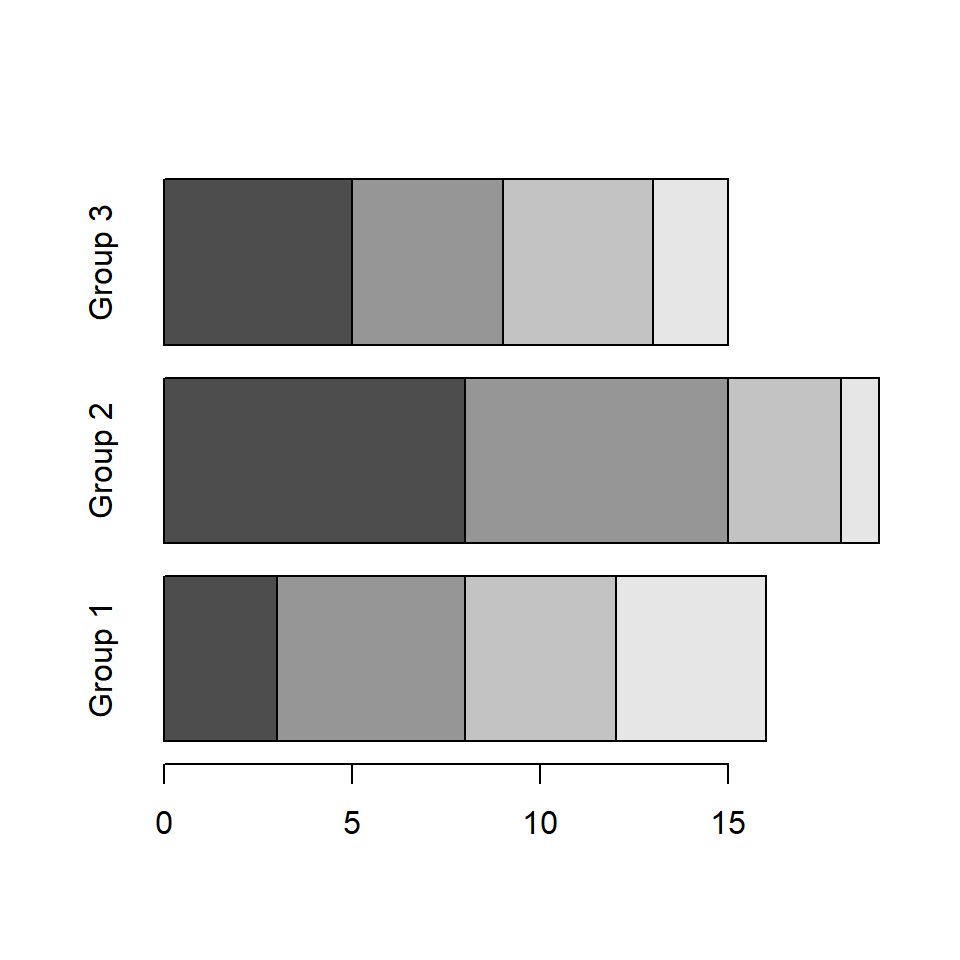Custom group names

The function also allows modifying the names of the groups displayed along the X-axis (Y-axis for horizontal bar plots) with `names.arg` argument.

``````barplot(tabl,
names.arg = c("G1", "G2", "G3"))``````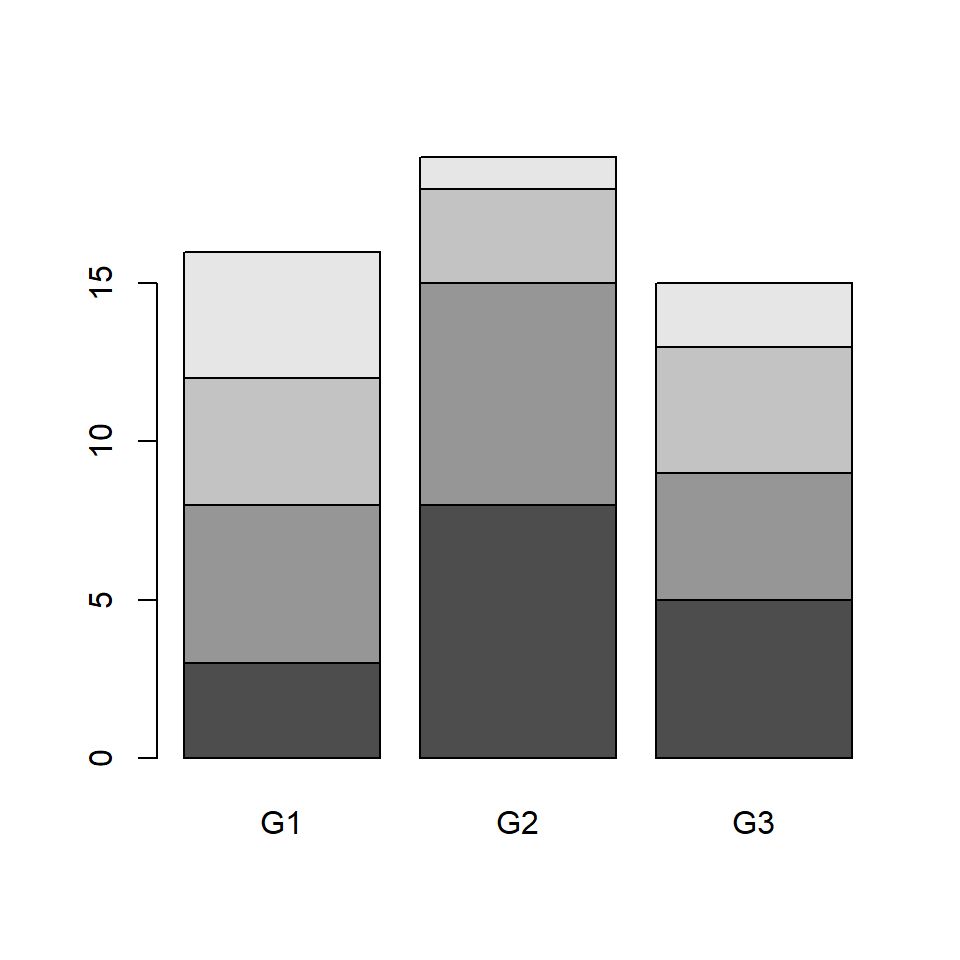### Fill color and borders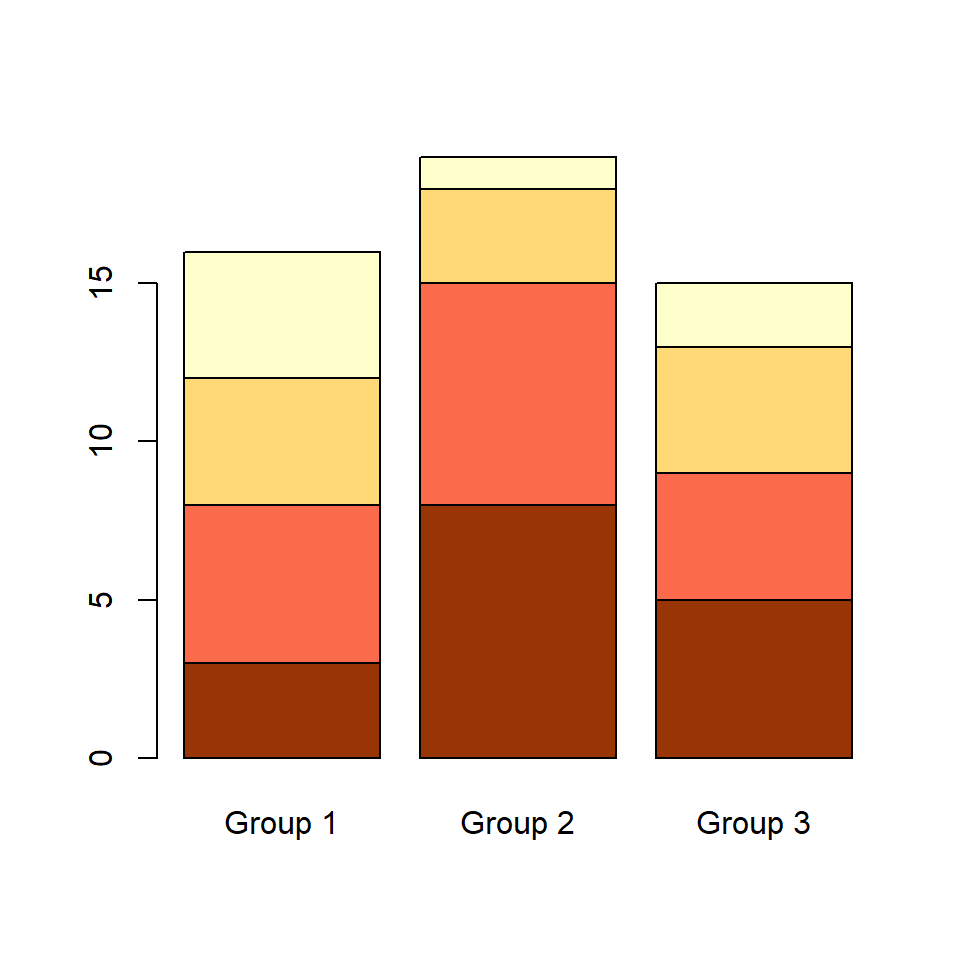Color palette

If you want to customize the color palette use `col` argument. You will need to specify as many colors as rows your table has. Check the color palette section to choose your palette.

``````barplot(tabl, col = c("#993404", "#FB6A4A",
"#FED976", "#FFFFCC"))``````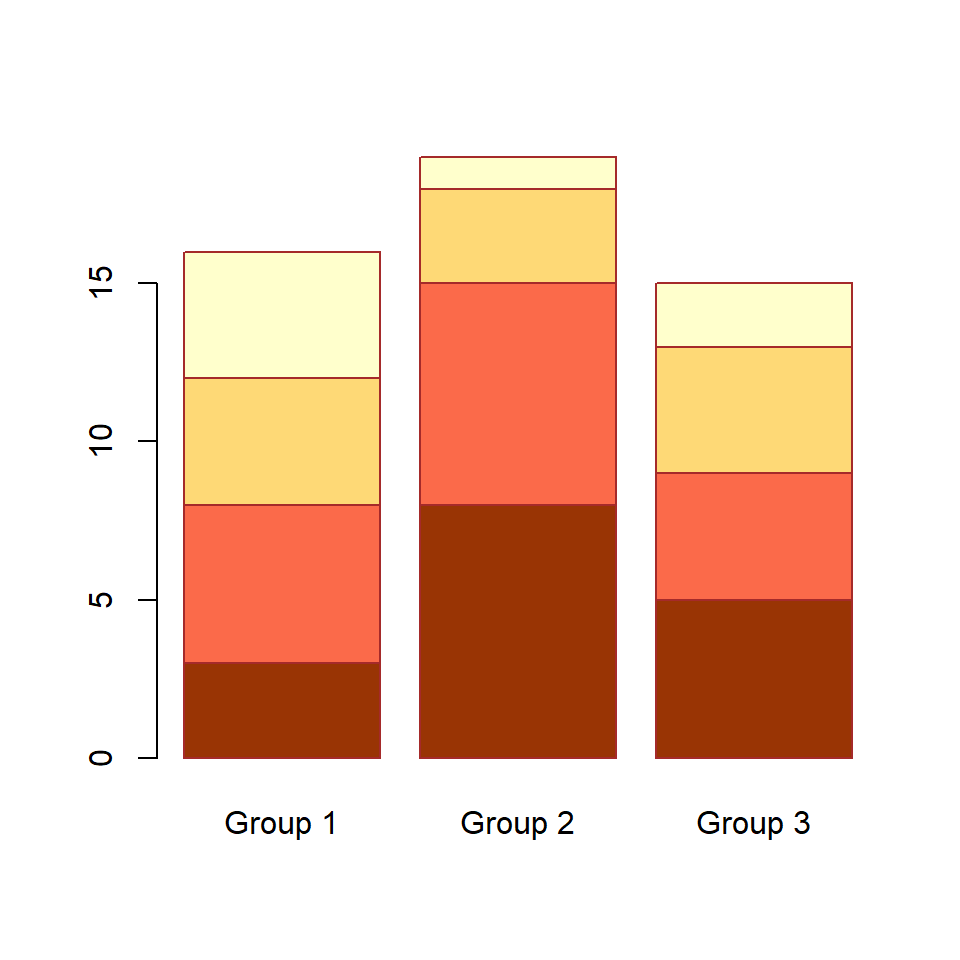Border color

The border color, which defaults to black, can be customized with `border` argument.

``````# Stacked bar plot with brown border
barplot(tabl, col = c("#993404", "#FB6A4A",
"#FED976", "#FFFFCC"),
border = "brown")``````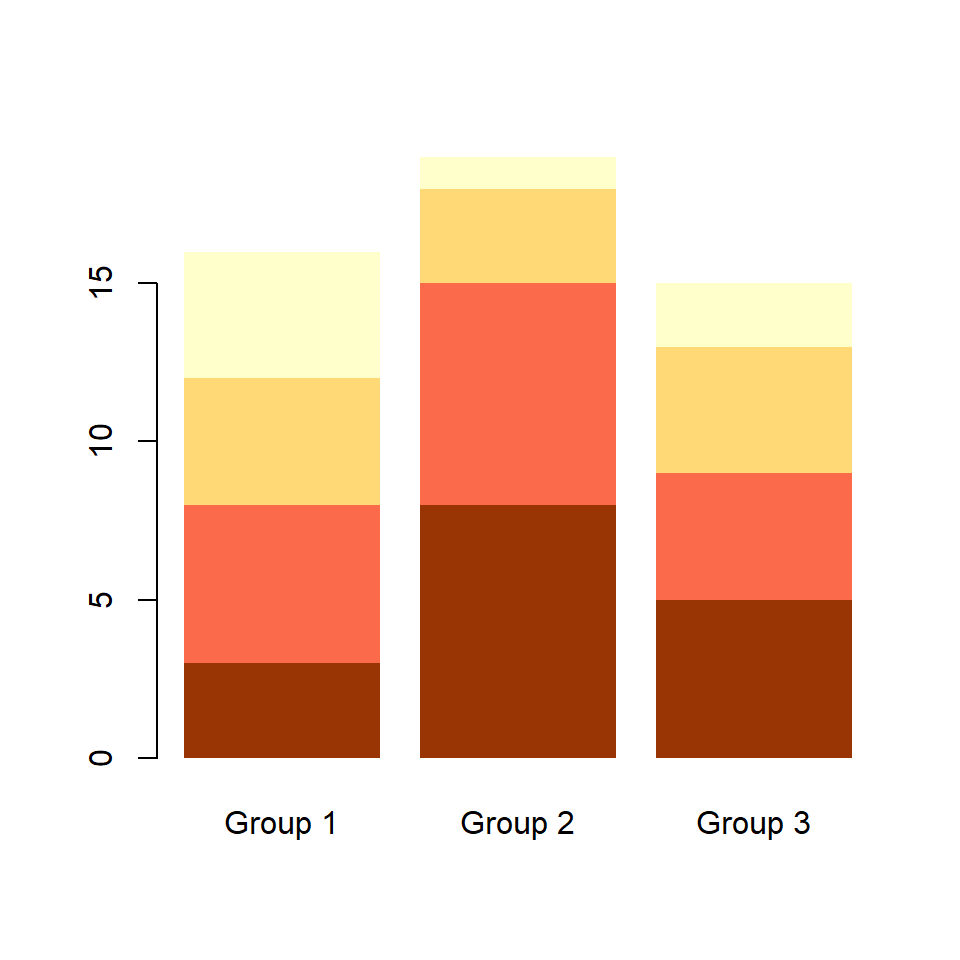Remove the borders

If you prefer to remove the all the borders set `border = NA`.

``````# Stacked bar plot without border
barplot(tabl, col = c("#993404", "#FB6A4A",
"#FED976", "#FFFFCC"),
border = NA)``````

### Adding a legend to the stacked plot

The `barplot` function comes with an argument named `legend.text`, which allows adding a legend to the bar graph without using the `legend` function.

``````# Stacked bar plot with legend
barplot(tabl, col = c("#993404", "#FB6A4A",
"#FED976", "#FFFFCC"),
legend.text = rownames(tabl))``````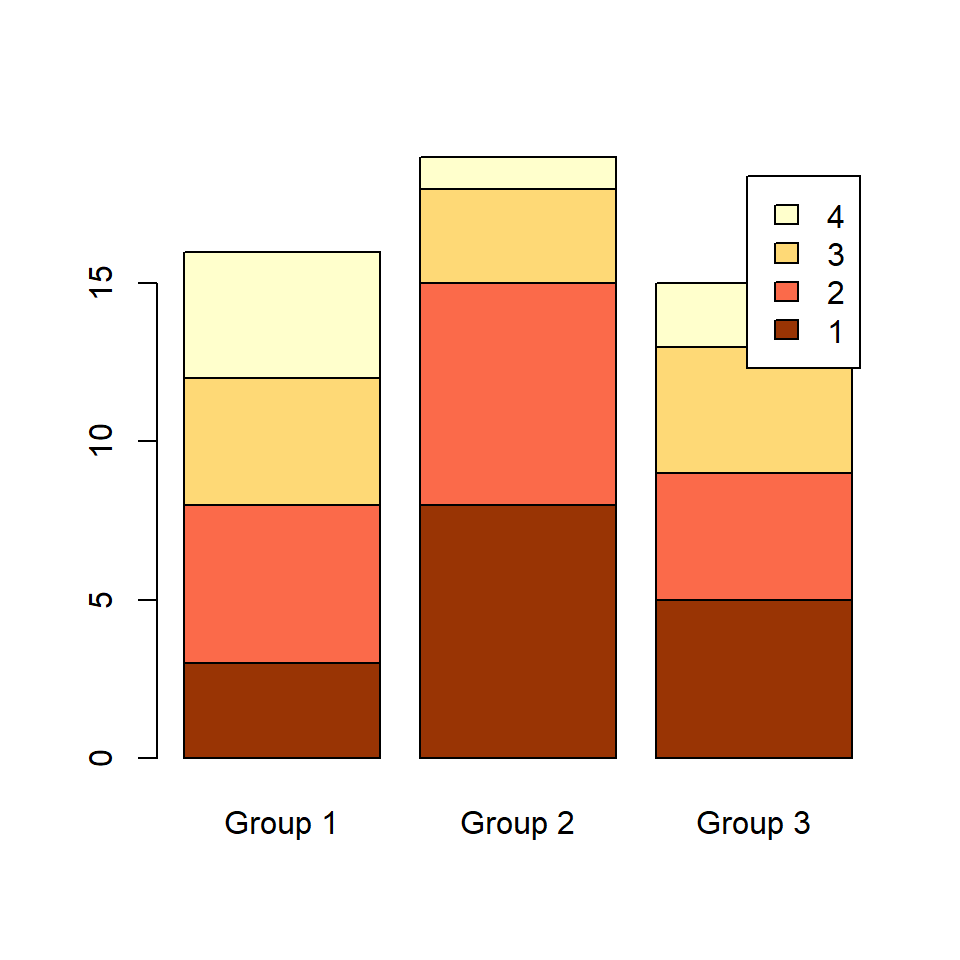Legend customization

If you want to customize the legend you can pass the arguments of the `legend` function to `args.legend` argument as a list.

``````# Stacked bar plot with legend
barplot(tabl, col = c("#993404", "#FB6A4A",
"#FED976", "#FFFFCC"),
legend.text = rownames(tabl),
args.legend = list(x = "topleft"))``````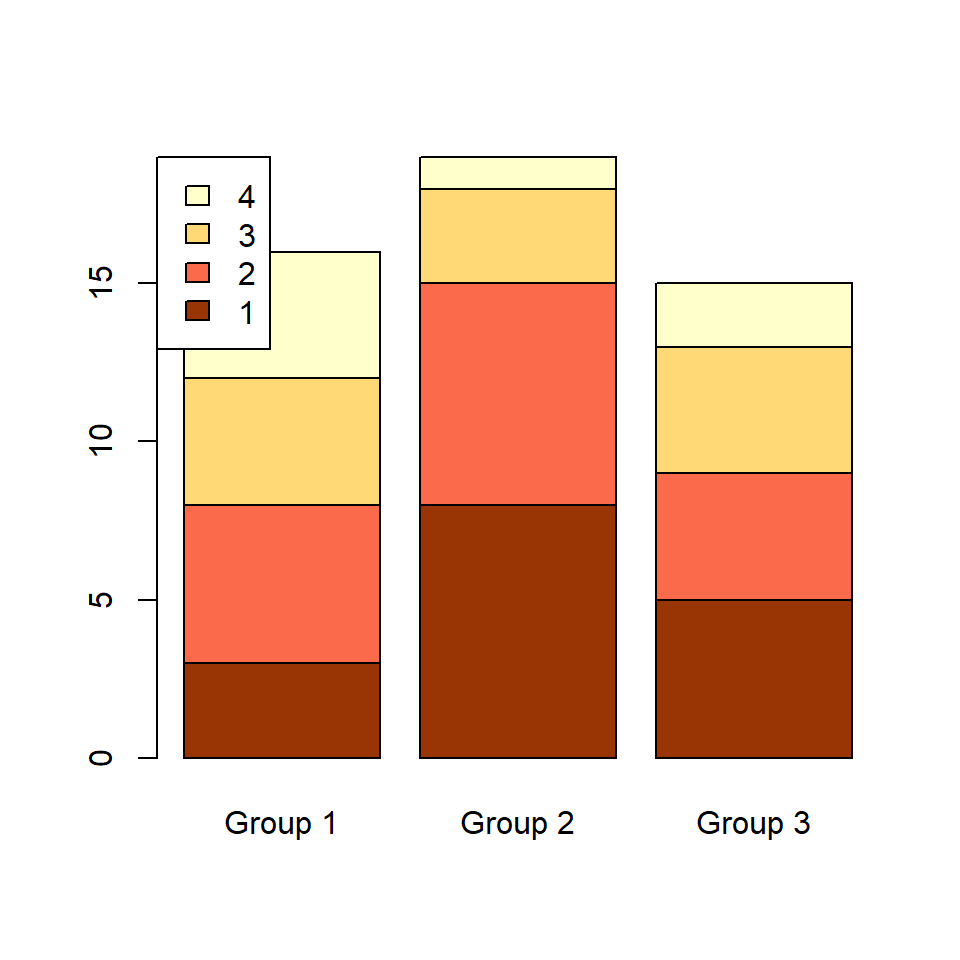Legend outside the bar chart

You may have noticed that the legend is over the bar chart in our example. If you want to place the legend outside the chart increase the corresponding margin and fine tune the position of the legend with `inset` as in the following example.

``````# Increase the right margin
par(mar = c(5.1, 4.1, 4.1, 4))

# Stacked bar chart with legend
barplot(tabl,
col = c("#993404", "#FB6A4A",
"#FED976", "#FFFFCC"),
legend.text = rownames(tabl),
args.legend = list(x = "topright",
inset = c(-0.2, 0))) ``````